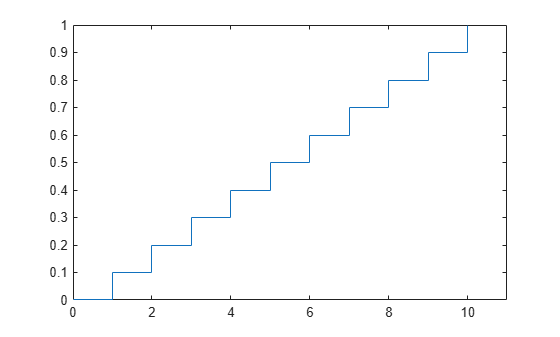## Uniform Distribution (Discrete)

### Definition

The discrete uniform pdf is

`$y=f\left(x|N\right)=\frac{1}{N}{I}_{\left(1,...,N\right)}\left(x\right)$`

### Background

The discrete uniform distribution is a simple distribution that puts equal weight on the integers from one to N.

### Examples

#### Plot a Discrete Uniform Distribution cdf

As for all discrete distributions, the cdf is a step function. The plot shows the discrete uniform cdf for N = 10.

```x = 0:10; y = unidcdf(x,10); figure; stairs(x,y) h = gca; h.XLim = [0 11];```#### Generate Discrete Uniform Random Numbers

Pick a random sample of 10 from a list of 553 items.

```rng default; % for reproducibility numbers = unidrnd(553,1,10)```
```numbers = 1×10 451 501 71 506 350 54 155 303 530 534 ```